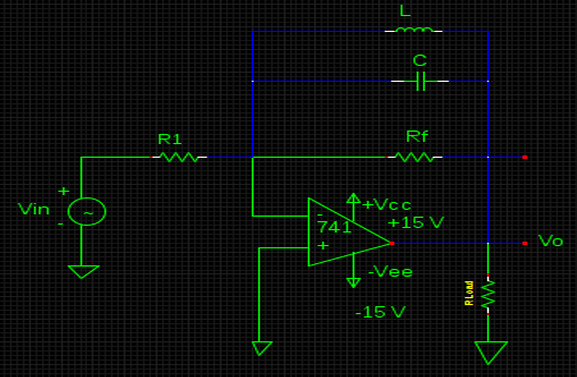# Numerical on AC non-inverting amplifier with single supply.

## What will be the values of bandwidth & maximum output voltage swing respectively in an non-inverting amplifier with input resistance of about 40 ohm, input capacitance of about 0.1 μ F with inclusive of R1=R2=R3=200 K Ω , Rf = 2 M Ω and Vcc= +20V?a. 32.56 kHz & 20 Vpp
b. 30.56 kHz & 20 Vpp
c. 47.56 kHz & 20 Vpp
d. 67.56 kHz & 20 Vpp

Correct Answer : c. 47.56 kHz & 20 Vpp

Explanation :

In accordance to given data, the ac input resistance of the amplifier can be given by,

Rif= (R2)¦(R3) ¦ [Ri (1+AB)]

When [Ri (1+AB)] >>R2 or R3 then, the above equation becomes

Rif ≈ (R2)¦(R3)
≈ 200K ¦ 200K
= 100K Ω
The low frequency cut-off limit can be exaggerated using

fL = 1 / [2 πCi(RiF + R0)]

=1 / [2 π (0.1 x 10 -6) (100K +40 )]
= 62.8 Hz
The gain of an amplifier is = 1+ (Rf / R1)
= 1 + ( 2M / 100K)
= 21

From the formula of Unity gain bandwidth,
UGB = Af x FH
Therefore, fH = UGB / Af = 1 M / 21 = 47.61 Khz

Bandwidth = FH – FL = 47.61 Khz – 0.0476 KHz = 47.56 KHz &
Ideal Maximum Output Voltage Swing = +Vcc = +20Vpp.